# Introduction

In this method, we find a technique for finding an interpolating polynomial which may be found in a simple-to-program manner, and allows fast, numerically-stable evaluation.

# Background

Useful background for this topic includes:

• Polynomials

# Theory

With Lagrange polynomials, we found the interpolating polynomial by taking each point xi and finding a polynomial yiLi(x) with the property that:

• yiLi(xi) = yi, and
• yiLi(xj) = 0 for ji.

This technique is the most easily implemented by humans (at least for linear and quadratic interpolating polynomials), but is also the least useful programatically. Also, if we found the Lagrange polynomial passing through the points (x1y1), ..., (xnyn), this gives us very little information for finding the Lagrange polynomial which passes through these n points together with a new point (xn + 1yn + 1).

Let us, however, use this idea: given n points, how do we find the polynomial which passes through the given n points and another point (xn + 1yn + 1)?

To demonstrate this, we will find the interpolating quadratic polynomial which passes through the three points in Figure 1.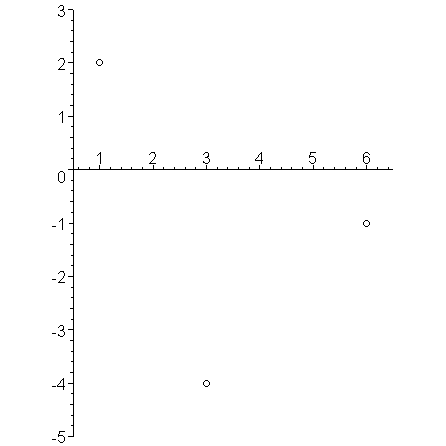Figure 1. Three points to be interpolate.

We will do this by finding a sequence of polynomials p0(x), p1(x), and p2(x) of degrees 0, 1, and 2 where the last will interpolate all three points.

### Constant (n = 1)

To do this, let us start from the beginning with a single point (x1, y1). The polynomial passing through this point is p0(x) = y1. For the sample points, p0(x) = 2 is shown in Figure 2.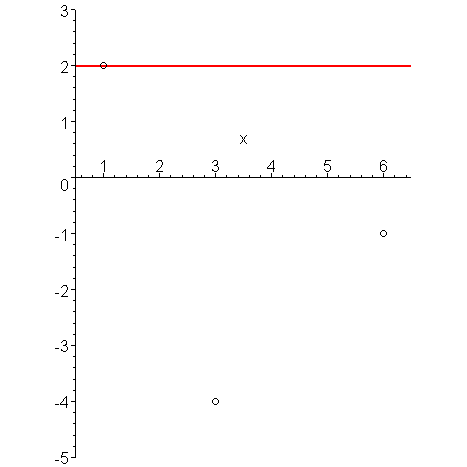Figure 2. Constant interpolant through the first point.

### Linear (n = 2)

Having found p0(x), we will find p1(x) by defining a polynomial Δp1(x) such that:

1. Δp1(x1) = 0
2. Δp1(x2) = y2 − p0(x2)

The first requirement makes it quite clear that the delta-polynomial must be of the form Δp1(x) = c2(xx1). We will use the second condition to determine c2:

c2(x2x1) = y2y1

Dividing through by x2x1, we get:

Given the example points, c2 = (-4 − 2)/(3 − 1) = -3, and Figure 3 shows p0(x) = 2 in blue, Δp1(x) = 2 − 3(x − 1) in magenta, and summing these together, we get that p1(x) passes through both the first and second points.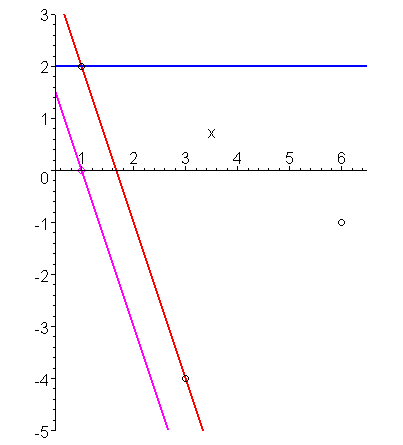Figure 3. Linear interpolant through the first and second points.

Given that the function p2(x) passes through two points, we want to find a function Δp2(x) such that p2(x) = p1(x) + Δp2(x) passes through all three points.

Again, to be zero at the first two points, Δp2(x) = c3(xx1)(xx2), and therefore we use p2(x3) = p1(x3) + Δp2(x3) = y3 to determine c3:

To simplify the algebra, we will add 0 = -y2 + y2 and 0 = x2x2 in two places in the right-hand side of this equation:

Isolating c3, we get:

The second and fourth terms cancel each other yeilding

By taking a common denominator, we get

Notice that the numerator is the difference between two

y21 = (y2 - y1)/(x2 - x1)
y32 = (y3 - y2)/(x3 - x2)

And finally, we could define:

y321 = (y32 - y21)/(x3 - x1)

Then we note that c1 = y1, c2 = y21, and c3 = y321; and therefore, the Newton interpolating polynomial is:

p2(x) = y1 + y21(x - x1) + y321(x - x1)(x - x2).

In general, if we define

then the interpolating Newton polynomial of degree n − 1 is

pn − 1(x) = y1 + y21(x − x1) + y321(x − x1)(x − x2) + ··· yn···321(x − x1)(x − x2)···(x − xn − 1)

# Problem

Find a Newton interpolating polynomial which passes through n points (x1, y1), ..., (xn, yn), that is, find the coefficients of the polynomial

# Assumptions

The x values are unique.

# Process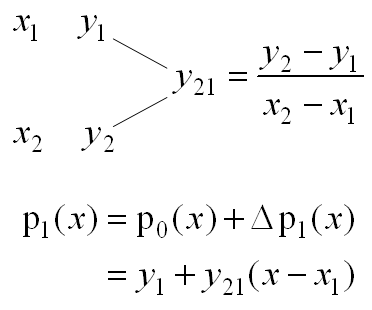Figure 4. Finding the interpolating linear polynomial.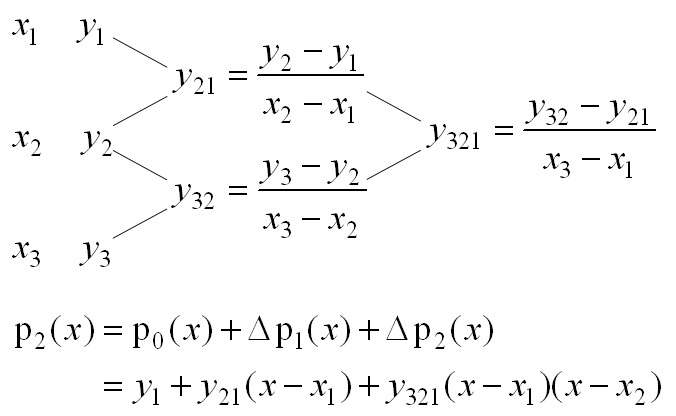Figure 5. Finding the interpolating quadratic polynomial.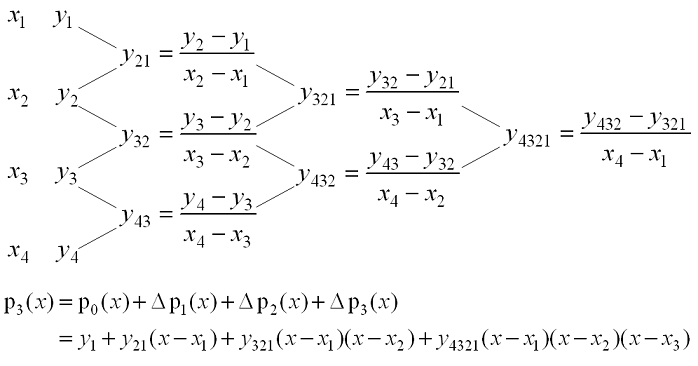Figure 6. Finding the interpolating cubic polynomial.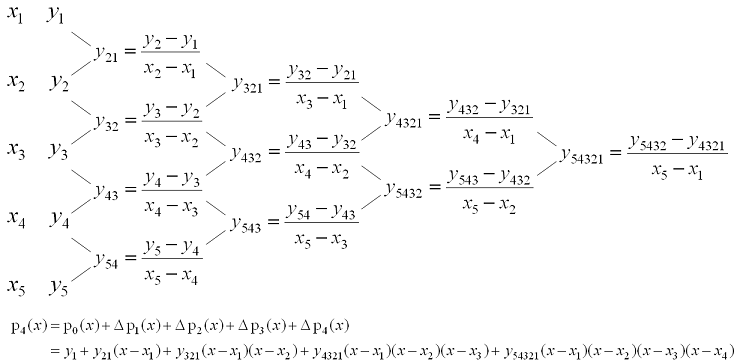Figure 7. Finding the interpolating quartic polynomial.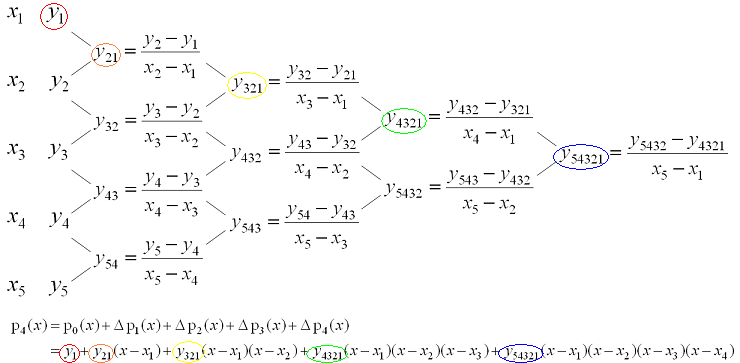Figure 8. Highlighted coefficients for the interpolating polynomial in Figure 7.

# Error Analysis

The error, in general is similar to that of the Vandermonde method, but it has better characteristics and is not subject to the same unknown catastrophic cancellation the Vandermonde method is subject to. The next topic on Horner's rule shows how to evaluate Newton polynomials.

# Examples

1. Find the Newton polynomial which interpolates the points (2, 2), (3, 1), (5, 2).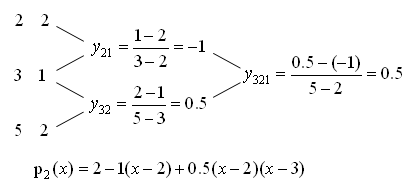Figure 9. The table for finding the Newton polynomial interpolating (2, 2), (3, 1), (5, 2).

Therefore, the interpolating polynomial is p2(x) = 2 − (x − 2) + 0.5 (x − 2)(x − 3).

2. Find the polynomial which interpolates the points (-2, -39), (0, 3), (1, 6), (3, 36).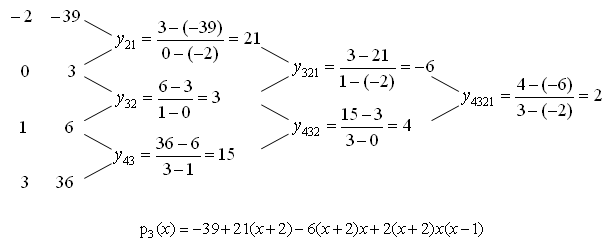Figure 10. The table for finding the Newton polynomial interpolating (-2, -39), (0, 3), (1, 6), (3, 36).

Therefore, the interpolating polynomial is p3(x) = -39 + 21 (x + 2) − 6 (x + 2)x + 2 (x + 2)x(x − 1).

3. Use Matlab to find the polynomial which interpolates the points (-3.2, 4.5), (-1.5, 0.5), (0.3, 0.6), (0.7, 1.2), (2.5, 3.5).

```>> x = [-3.2, -1.5, 0.3, 0.7, 2.5]';
>> y = [ 4.5,  0.5, 0.6, 1.2, 3.5]';
>> p = y;
>> for i = 1:(length(x) - 1)
p( (i + 1):end ) = (p( (i + 1):end ) - p( i:(end - 1) )) ./ (x( (i + 1):end ) - x( 1:(end - i) ));
end
p
```

4. Why are the memory requirements of the code in Example 3 O(n)?

Only three arrays of size n = 5 and one of size n = 4 are allocated. All other computations are done using already existing entries in the vector p.

5 Suppose we have found the Newton polynomial which interpolates the points (1, 4), (3, -2), (4, 10), (5, 16) using the triangle:

```        1     4
-3
3    -2           5
12          -2
4    10          -3
6
5    16
```

Use this table to calculate the interpolating polynomial passing through the points (3, -2), (4, 10), (5, 16), (7, 34) using the least possible number of differences and divisions.

In this case, we already have part of the tree:

```
3    -2
12
4    10          -3
6
5    16

7    34
```

Therefore, we only need fill in the remaining 3 entries:

```
3    -2
12
4    10          -3
6           1
5    16            1
9
7    34
```

Thus, the interpolating polynomial is p(x) = -2 + 12(x - 3) - 3(x - 3)(x - 4) + (x - 3)(x - 4)(x - 5).

# Question 1

Find the Newton polynomial which interpolates the points (2, 3) and (5, 7).

Answer: p(x) = 3 + 4/3 (x - 2)

# Question 2

Find the Newton polynomial which interpolates the points (-2, 21), (0, 1), (1, 0), (3, -74).

Answer: p(x) = 21 - 10(x + 2) + 3(x + 2)x - 3(x + 2)x(x - 1).

# Question 3

Find the Newton polynomial which interpolates the points (1, 5), (2, 7), (4, 11), (6, 15).

Answer: p(x) = 5 + 2(x - 1)

# Question 4

Use Matlab to find the Newton polynomial which interpolates the points

(1.3, 0.51), (0.57, 0.98), (-0.33, 1.2), (-1.2, 14), (2.1, -0.35), (0.36, 0.52)

Answer: The coefficients are 0.51, -0.6438, -0.2450, -3.3677, 1.0159, 0.8223.

# Question 5

Must the x values be ordered from smallest to largest for the method to find Newton polynomials to work?

# Applications to Engineering

Newton polynomials provide a technique which allows an interpolating polynomial of n points to be found in O(n2) time but only O(n) space. Horner's rule provides a very efficient method of evaluating these polynomials.

# Matlab

Newton interpolating polynomial may be found easily in Matlab:

```>> x = [1.2 1.5 1.9 2.5 2.6]';
>> y = [0.5 0.3 0.5 0.2 0.7]';
>> ys = y;
>> for i=2:length(ys)
ys(i:end) = (ys(i:end) - ys((i - 1):(end - 1))) ./ (x(i:end) - x(1:end - i + 1));
end
>> ys
ys =

0.50000
-0.66667
1.66667
-2.05128
7.21659
```

This creates a vector containing the coefficients of the Newton polynomial.

We could replace the body of the for loop with the shorter version using the diff statement:

```>> for i=2:length(ys)
ys(i:end) = diff( ys(i - 1:end) ) ./ (x(i:end) - x(1:end - i + 1));
end
```

# Maple

Finding an interpolating polynomial with Maple may be done with the PolynomialInterpolation routine:

```CurveFitting[PolynomialInterpolation]( [[0.3, 1.5], [0.4, 1.7], [0.6, 2.5]], x, form=Newton );
plot( %, x = 0..1 );
```

For more help on either of this routine or on the CurveFitting package, enter:

```?CurveFitting
?CurveFitting,PolynomialInterpolation
```

# BLAS

The basic linear algebra subroutine (BLAS) for for finding the coefficients of the Newton polynomial is

```  int gsl_poly_dd_init( double dd[], double xs[], double ys[], int N );
```

This example finds the coefficients of the Newton polynomial interpolating the stated points

```#include <stdio.h>
#include <gsl/gsl_poly.h>

/*************************************************
* Find the Newton polynomial coefficients
*************************************************/

#define N 5

int main() {
/* The points
(0.1, 1.2), (0.5, 2.7), (0.7, 3.8), (1.2, 4.7), (1.5, 6.0) */

double xs[N] = {0.1, 0.5, 0.7, 1.2, 1.5};
double ys[N] = {1.2, 2.7, 3.8, 4.7, 6.0};

/* The coefficients of the newton polynomial. */
double dd[N];
gsl_poly_dd_init( dd, xs, ys, N );

int i;

for ( i = 0; i < N; ++i ) {
printf( "%5.10lf  ", dd[i] );
}

printf( "\n" );

return 0;
}
```

To compile and execute this code, you must have the GNU Scientific Library installed on your system, either in cygwin or in Linux. The example below looks at how you compile this code in Cygwin:

```\$ gcc -Wall -I/usr/include newton.c -lgsl -lgslcblas -lm
\$ ./a.exe
```

The output is

```1.2000000000  3.7500000000  2.9166666667  -7.4567099567  11.3636363636
```

This can be seen to be the correct result by calculating the divided difference table:

```0.1    1.2
3.7500
0.5    2.7             2.9167
5.5000             -7.4567
0.7    3.8            -5.2857              11.364
1.8000              8.4524
1.2    4.7             3.1667
4.3333
1.5    6.0
```